## Pages

SUBSCRIBE HERE TO GET POST UPDATES VIA EMAIL :

Delivered by FeedBurner

# Data types - Basic Java Tutorials For Selenium WebDriver

I have received many requests from my blog readers for posting some basic java tutorials which are really required in selenium webdriver software testing process. So now I have planned to post some basic java tutorial posts which are really required in selenium webdriver learning and implementation in your software testing process. These tutorials will help you for selenium webdriver interview preparation too. Let we start from different data types In java which we can use in our selenium webdriver software test case preparation.

In java or any other programming languages, data types represents that which type of values can be stored and what will be the size of that value or how much memory will be allocated. There are nearest eight different data types in java like byte, short, int, long, float, double, char, Boolean. byte and short datatypes are not much more useful in selenium webdriver so I am skipping them here to decrease your confusions.

int datatype
int data type is useful to store 32 bit integer (Example : 4523) values only. We can not store decimal (Example 452.23) values in int data type.
Example :
``int i = 4523;``

long datatype
long datatype is useful to store 64 bit integer(Example : 652345) values. You can use it when your value is more larger and can not hold it in int. Same as int datatype, we can not store decimal (Example 452.23) values in long datatype
Example :
``long l = 652345;``

double datatype

double datatype is useful to store 64 bit decimal(Example : 56.2354) values. We can store integer (Example 12456) values too in double datatype.
Example :
``````double d1 = 56.2354;
double d2 = 12456;``````

char datatype

char datatype is useful to store single character(Example : 'd'). It can not store more than one (Example 'dd') character in it.
Example :
``char c = 'd';``

boolean datatype
boolean datatype is useful to store only boolean(Example : true) values.
Example :
``boolean b = true;``

String Class
String is not a data type but it is a class which is useful to store string in variable.
Example :
``String str = "Hello World";``

VIEW DIFFERENT FUNCTIONS OF STRING CLASS

Created bellow given example for all these datatypes. Run it in your eclipse and verify results.
``````public class datatypes {

public static void main(String[] args) {
int i = 4523;   //Can store 32 bit integer values only.
long l = 652345;  //Can store 64 bit integer values only.
double d1 = 56.2354;         //Can store 64 bit decimal values.
double d2 = 12456;  //We can use it for integer values too.
char c = 'd';   //Can store single character only.
boolean t = true;  //Can store only boolean values like true or false.
String str = "Hello World";  //Can store any string values.

System.out.println("Integer Var Is --> "+i);
System.out.println("Long Var Is --> "+l);
System.out.println("double Var d1 Is --> "+d1);
System.out.println("double Var d2 Is --> "+d2);
System.out.println("char Var c Is --> "+c);
System.out.println("boolean Var b Is --> "+t);
System.out.println("boolean Var str Is --> "+str);
}
}``````

Bellow given result will display in your console at the end of execution.
``````Integer Var Is --> 4523
Long Var Is --> 652345
double Var d1 Is --> 56.2354
double Var d2 Is --> 12456.0
char Var c Is --> d
boolean Var b Is --> true
String Var str Is --> Hello World``````

1.2.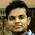3.4.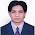Good tutorial.

5.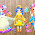Greate Stuff...

6.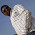7.Very clear and perfect.....

8.9.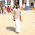10.11.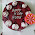12.13.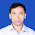14.15.easy to Bigginners,,and thanks to upload

16.17.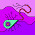Like the introduction ..Hope I will be able to learn as easy as this :)

18.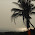Very useful blog...Thanks...

19.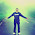great..thanks

20.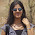Easy to learn for beginners..Nice..

21.Easy to understand

22.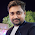its really useful and easy to learn.... great !!!

23.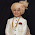24.Good work.. Thank you... Very useful and easily understandable..

1.Many thanks for great tutorials.

25.26.float is also considered as one of the primitive DataTypes in java

27.Good work sir...Thank you

28.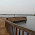I liked the tutorial, thanks

29.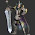It is good, thank you

30.Very useful for me Thank you so much :)

31.Very useful to me thank you so much

32.Hey thanks for the tutorial, there is a small mistake in this statement "System.out.println("boolean Var str Is --> "+str); ---- boolean should reflect as String.

1.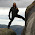Yes, last line should be: System.out.println("String Var str Is" + str);

2.33.Thank you,

34.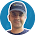It would be great if you can include the minimum and maximum values that can be stored in various data types for example -66536 to 66536.

35.It's very easy to understand and helpful for beggineers

36.Thank you so much...

37.Vey basic and useful information

38.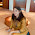very basic and useful information

39.nice one sir ji..carry on we except more,.....thansk for your valuable time

40.nice article com blog..thanks for your valuable time, it really help me ..

41.It would be great..Thank you so much.

42.SUPER! Thank YOu!